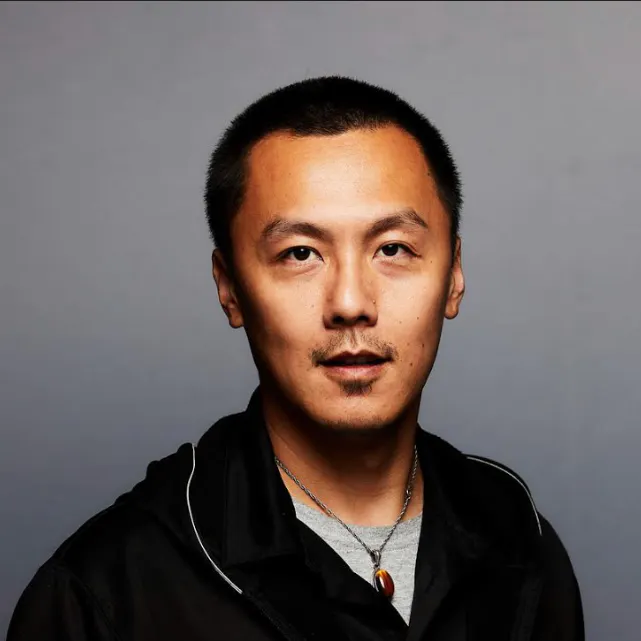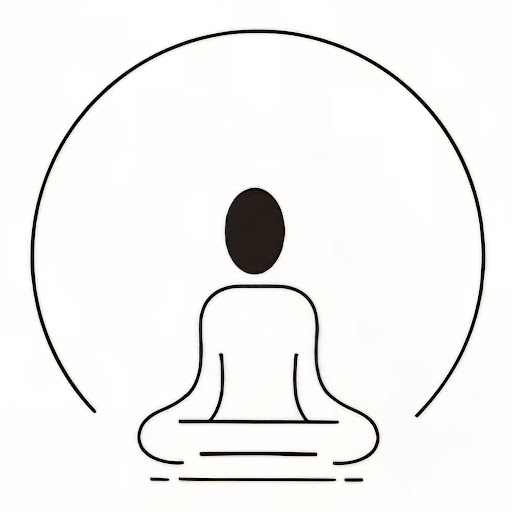# Guardian Camera AI

Using TensorFlow AI to detect fall for cameras

IntermediateFull instructions provided20 hours2,491

## Things used in this project

### Hardware components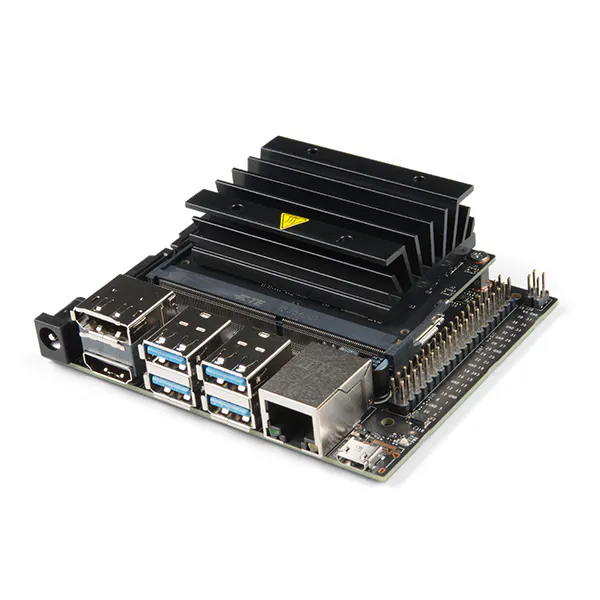NVIDIA Jetson Nano Developer Kit
×1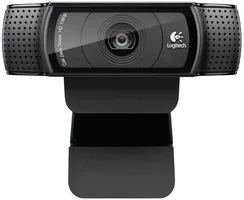Webcam, Logitech® HD Pro
×1

### Software apps and online services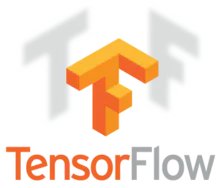TensorFlow

## Schematics

### Jetson Diagram

Jetson diagram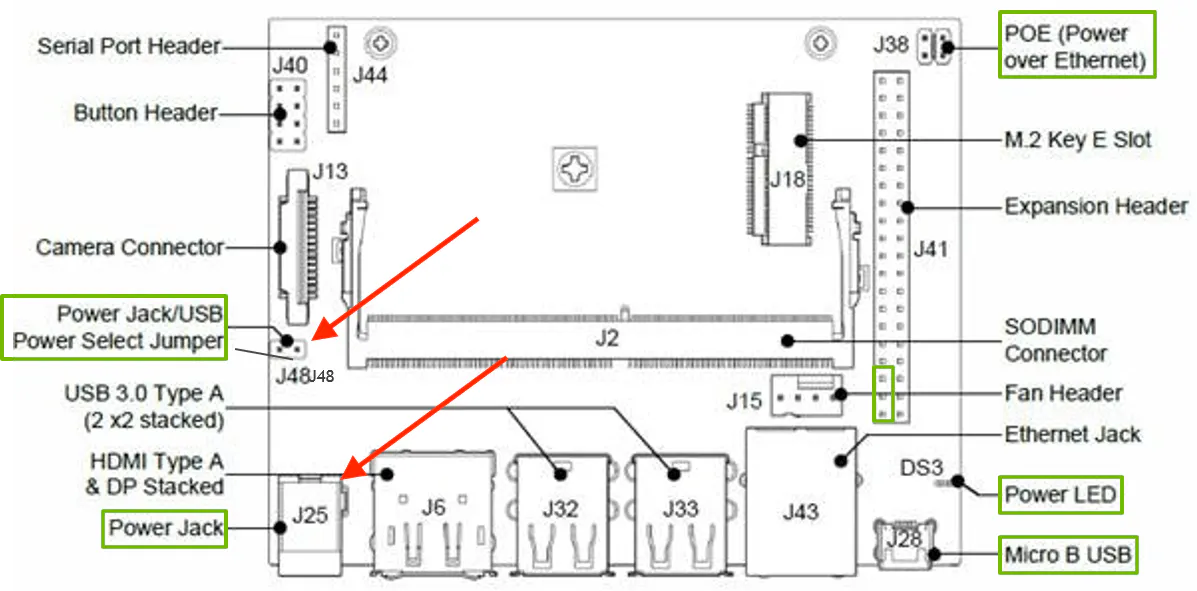## Code

### fall.py

Python
Python fall code with darknet
```from ctypes import *
import cv2
import sys
sys.path.append('python')
import darknet as dn
import time
import math
import random

def sample(probs):
s = sum(probs)
probs = [a/s for a in probs]
r = random.uniform(0, 1)
for i in range(len(probs)):
r = r - probs[i]
if r <= 0:
return i
return len(probs)-1

def c_array(ctype, values):
arr = (ctype*len(values))()
arr[:] = values
return arr

class BOX(Structure):
_fields_ = [("x", c_float),
("y", c_float),
("w", c_float),
("h", c_float)]

class DETECTION(Structure):
_fields_ = [("bbox", BOX),
("classes", c_int),
("prob", POINTER(c_float)),
("objectness", c_float),
("sort_class", c_int)]

class IMAGE(Structure):
_fields_ = [("w", c_int),
("h", c_int),
("c", c_int),
("data", POINTER(c_float))]

_fields_ = [("classes", c_int),
("names", POINTER(c_char_p))]

lib = CDLL("/home/ai/workspace/darknet/libdarknet.so", RTLD_GLOBAL)
lib.network_width.argtypes = [c_void_p]
lib.network_width.restype = c_int
lib.network_height.argtypes = [c_void_p]
lib.network_height.restype = c_int

predict = lib.network_predict
predict.argtypes = [c_void_p, POINTER(c_float)]
predict.restype = POINTER(c_float)

set_gpu = lib.cuda_set_device
set_gpu.argtypes = [c_int]

make_image = lib.make_image
make_image.argtypes = [c_int, c_int, c_int]
make_image.restype = IMAGE

get_network_boxes = lib.get_network_boxes
get_network_boxes.argtypes = [c_void_p, c_int, c_int, c_float, c_float, POINTER(c_int), c_int, POINTER(c_int)]
get_network_boxes.restype = POINTER(DETECTION)

make_network_boxes = lib.make_network_boxes
make_network_boxes.argtypes = [c_void_p]
make_network_boxes.restype = POINTER(DETECTION)

free_detections = lib.free_detections
free_detections.argtypes = [POINTER(DETECTION), c_int]

free_ptrs = lib.free_ptrs
free_ptrs.argtypes = [POINTER(c_void_p), c_int]

network_predict = lib.network_predict
network_predict.argtypes = [c_void_p, POINTER(c_float)]

reset_rnn = lib.reset_rnn
reset_rnn.argtypes = [c_void_p]

do_nms_obj = lib.do_nms_obj
do_nms_obj.argtypes = [POINTER(DETECTION), c_int, c_int, c_float]

do_nms_sort = lib.do_nms_sort
do_nms_sort.argtypes = [POINTER(DETECTION), c_int, c_int, c_float]

free_image = lib.free_image
free_image.argtypes = [IMAGE]

letterbox_image = lib.letterbox_image
letterbox_image.argtypes = [IMAGE, c_int, c_int]
letterbox_image.restype = IMAGE

rgbgr_image = lib.rgbgr_image
rgbgr_image.argtypes = [IMAGE]

predict_image = lib.network_predict_image
predict_image.argtypes = [c_void_p, IMAGE]
predict_image.restype = POINTER(c_float)

def array_to_image(arr):
arr = arr.transpose(2,0,1)
c = arr.shape
h = arr.shape
w = arr.shape
arr = (arr/255.0).flatten()
data = dn.c_array(dn.c_float, arr)
im = dn.IMAGE(w,h,c,data)
return im

def detect(net, meta, image, thresh=.24, hier_thresh=.5, nms=.45):
num = c_int(0)
pnum = pointer(num)
predict_image(net, im)
dets = get_network_boxes(net, im.w, im.h, thresh, hier_thresh, None, 0, pnum)
num = pnum
if (nms): do_nms_obj(dets, num, meta.classes, nms);

res = []
for j in range(num):
for i in range(meta.classes):
if dets[j].prob[i] > 0:
b = dets[j].bbox
res.append((meta.names[i], dets[j].prob[i], (b.x, b.y, b.w, b.h)))
res = sorted(res, key=lambda x: -x)
free_image(im)
free_detections(dets, num)
return res

def isFall(w,h):
if float(w)/h>=1.1:
return True
else:
return False

#open the input video file
cap=cv2.VideoCapture(0)

res=[]
frame_number=0
while True:
# Grab a single frame of video
frame_number += 1

# Quit when the input video file ends
if not ret:
break
'''
# detect per 2 frame
if frame_number%2==0:
continue
'''
# append all the coordinate of the detected person to res
#    im = array_to_image(frame)
start=time.time()
cv2.imshow('Fall detection',frame)
cv2.imwrite('check.jpg',frame)

r = detect(net, meta, 'check.jpg')
#print('the whole running time is: '+str(time.time()-start))
res=[]
for item in r:
if item=='person' or item=='dog' or item=='cat' or item=='horse':
res.append(item)
# if multiple exist, and there also contains person,  preserve person only!
#print('--------------')
#print(res)
if len(res)>1:
for item in res:
if item=='person':
res=[]
res.append(item)
break

# get the max rectangle
result=[]
maxArea=0
if len(res)>1:
for item in res:
if item*item>maxArea:
maxArea=item*item
result=item
elif len(res)==1:
result=res
#draw the result
if(len(result)>0):
print(result)
# label the result
left=int(result-result/2)
top=int(result-result/2)
right=int(result+result/2)
bottom=int(result+result/2)

#whether fall?
if isFall(result,result):
cv2.rectangle(frame, (left, top), (right, bottom), (0, 0, 255), 2)

# Draw a label with a name below the face
cv2.rectangle(frame, (left, bottom - 25), (right, bottom), (0, 0, 255))
font = cv2.FONT_HERSHEY_DUPLEX
cv2.putText(frame, 'Warning!!!', (left + 6, bottom - 6), font, 0.5, (255, 0, 0), 1)
else:
cv2.rectangle(frame, (left, top), (right, bottom), (255, 0, 0), 2)
cv2.imshow('Fall detection',frame)

# Hit 'q' on the keyboard to quit!
if cv2.waitKey(1) & 0xFF == ord('q'):
break
# All done!
cap.release()
cv2.destroyAllWindows()
```

## Credits

### Peter Ma

44 projects • 293 followers
Prototype Hacker, Hackathon Goer, World Traveler, Ecological balancer, integrationist, technologist, futurist.

### John Wan

0 projects • 1 follower

### Michael Wan

0 projects • 3 followers

### Natka Wojcik

1 project • 2 followers